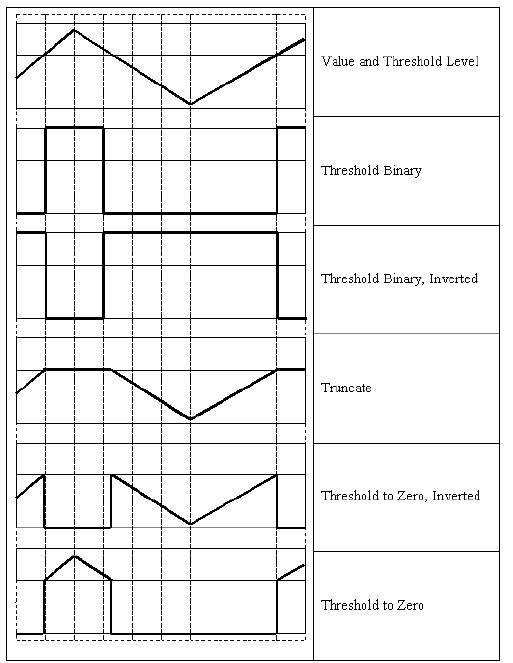# enum cv::ThresholdTypes

## Overview

type of the threshold operation

#include <imgproc.hpp>

enum ThresholdTypes
{
THRESH_BINARY     = 0,
THRESH_BINARY_INV = 1,
THRESH_TRUNC      = 2,
THRESH_TOZERO     = 3,
THRESH_TOZERO_INV = 4,
THRESH_OTSU       = 8,
THRESH_TRIANGLE   = 16,
};


## Detailed Documentation

type of the threshold operation### Enum Values

THRESH_BINARY

$\texttt{dst} (x,y) = \fork{\texttt{maxval}}{if $$\texttt{src}(x,y) > \texttt{thresh}$$}{0}{otherwise}$
THRESH_BINARY_INV

$\texttt{dst} (x,y) = \fork{0}{if $$\texttt{src}(x,y) > \texttt{thresh}$$}{\texttt{maxval}}{otherwise}$
THRESH_TRUNC

$\texttt{dst} (x,y) = \fork{\texttt{threshold}}{if $$\texttt{src}(x,y) > \texttt{thresh}$$}{\texttt{src}(x,y)}{otherwise}$
THRESH_TOZERO

$\texttt{dst} (x,y) = \fork{\texttt{src}(x,y)}{if $$\texttt{src}(x,y) > \texttt{thresh}$$}{0}{otherwise}$
THRESH_TOZERO_INV

$\texttt{dst} (x,y) = \fork{0}{if $$\texttt{src}(x,y) > \texttt{thresh}$$}{\texttt{src}(x,y)}{otherwise}$
THRESH_OTSU


flag, use Otsu algorithm to choose the optimal threshold value

THRESH_TRIANGLE


flag, use Triangle algorithm to choose the optimal threshold value